# C 402: Cracking a Short RSA Key (20 pts + 30 extra)

What you need:
• Any computer with Python 3.

## Purpose

To break into RSA encryption without prior knowledge of the private key. This is only possible for small RSA keys, which is why RSA keys should be long for security.

## Summary

Here's a diagram from the textbook showing the RSA calculations.## Problem Statement

Meghan's public key is (10142789312725007, 5). Find her private key.

## Installing pycryptodome

We'll need the pycryptodome library. To install it, execute this command:
``` python3 -m pip install pycryptodome ```

## Factoring n

### Finding the Square Root of n

n = 10142789312725007. This is the product of two prime numbers, p and q.

How large are p and q? Well, they can't both be larger than the square root of n, or they'd be larger than n when multiplied together.

Start Python 3 in interactive mode with this command:

``` python3 ```
Execute these commands:
```import math n = 10142789312725007 print(math.sqrt(n)) ```
The square root prints out, as shown below.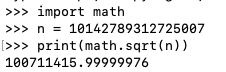### Testing 20 Candidates

So one of the prime factors must be a prime number less than 100711415. All we have to do is try dividing n by odd numbers starting at 100711413 and going down until we get an integer result. (We don't need to test 100711415 because it's divisible by 5 and therefore not a prime number.)

A good way to do this is to calculate n mod c, where c is a candidate. If c is a factor of n, the result will be zero.

We can test the first 20 candidates with a for loop.

Execute these commands:

```c = 100711413 for i in range(c, c-40, -2): print(i, n%i) ```
Press Enter twice after the last command to terminate the loop.

The third candidate is the winner, with a remainder of zero, as shown below.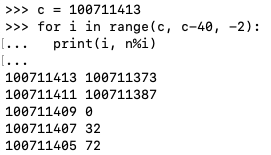### Calculating q

We now know p and we can calculate q.

Execute these commands:

```p = 100711409 q = int(n / p) print(p, q, n, p*q, n - p*q) ```
The calculation worked, so the last value is zero, as shown below.## Computing phin = (p-1) * (q-1)

Execute these commands:
```phin = (p-1) * (q-1) print(p, q, n, phin) ```
The parameters print out, as shown below.## Computing the Private Key d

We need to find a d with this property:
(d * e) mod phin = 1
d is the "multiplicative inverse" of e.

We know that e = 5 from the Problem Statement.

It's not obvious how to find d, but there's a simple way to do it in Python, using the "pycryptodome" library.

In the Terminal window running python, execute these commands.

```e = 5 from Crypto.Util.number import inverse d = inverse(e, phin) print(d, e, d*e %phin) ```
We get the value of d, and, to verify it, we see that d*e %phin is indeed 1, as shown below.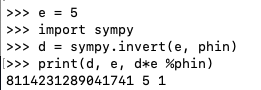## 5. Encrypting a Message

### Encoding the Message as a Number

Cueball wants to send Meghan this message:
```Hi! ```
We can only send numbers. Let's convert that message to three bytes of ASCII and then interpret it as a 24-bit binary value.

In the Terminal window running python, execute these commands.

```x1 = ord('H') x2 = ord('i') x3 = ord('!') x = x1*256*256 + x2*256 + x3 print(x) ```
We get the value of x, as shown below.### Encrypting the Message with the Public Key

A public key contains two numbers: n and e. To encrypt a message x, use this formula:Execute these commands:

```y = x ** e % n print(y) ```
The encrypted message appears, as shown below.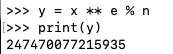## 6. Decrypting a Message

To decrypt a message, use this formula:Execute this command:
```xx = y ** d % n ```
Python freezes. It's going to take forever to calculate a number that large.

Press Ctrl+C to stop the computation.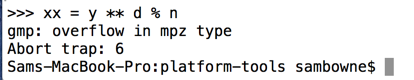To compute such a number, we must use the pow() function. Execute this command:
```xx = pow(y, d, n) print(xx) ```
It works, showing our original message in numerical form.### Converting the Message to Readable Text

To convert that number back to letters, execute these commands:
```x1 = int(xx / (256*256)) x2 = int((xx - 256*256*x1) / 256) x3 = int(xx - 256*256*x1 - 256*x2) msg = chr(x1) + chr(x2) + chr(x3) print(x1, x2, x3, msg) ```
Now it works, showing the original message in readable form, as shown below.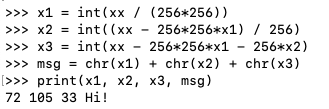# C 402.1: Encrypt "WOW" (10 pts)

Execute these commands to load the same keys used above:
```n = 10142789312725007
p = 100711409
q = int(n / p)
phin = (p-1) * (q-1)
e = 5
from Crypto.Util.number import inverse
d = inverse(e, phin)
```
Using those keys, encrypt this message:
```WOW
```
Hint 1: The message, converted to a decimal number, is 7 digits long and ends in 43.

Hint 2: The encrypted message is 16 digits long and ends in 66.

The flag is the encrypted message.

# C 402.2: Encrypt "OBEY!" (10 pts)

Using the same keys, encrypt this message:
```OBEY!
```
Hint 1: The message, converted to a decimal number, is 12 digits long and ends in 41.

Hint 2: The encrypted message is 16 digits long and ends in 81.

The flag is the encrypted message.

# C 402.3: Message to Cueball (15 pts)

Cueball's public key is:
```(111036975342601848755221, 13)
```
Meghan sends this message to Cueball. Decrypt it.
```80564890594461648564443
```
The flag is the decrypted message.

# C 402.4: Message to Rob (15 pts)

Rob public key is:
```(1234592592962967901296297037045679133590224789902207663928489902170626521926687, 5557)
```
Meghan sends this message to Rob. Decrypt it.
```272495530567010327943798078794037733865151017104532777645776936985235709526002
```
Hint: Make square root calculations more precise.

The flag is the decrypted message.

## Sources

Cracking short RSA keys